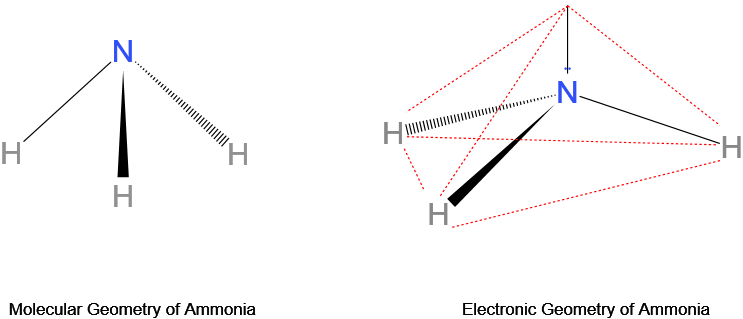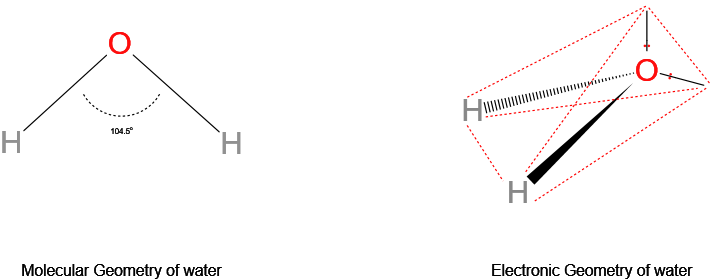Geometry in chemistry refers to the shape of molecules in 3-Dimensional space.

Molecular geometry is described as the 3D arrangement of atoms in a molecule, normally relative to a single central atom. Whereas, electron geometry is the 3D arrangement of electron pairs around a central atom, whether bonding or non-bonding.

A lone (non-bonding) pair refers to a pair of valence electrons that are not shared with another atom in a covalent bond, while a bond pair is a pair of electrons present in a bond.

It is well known that the electron pairs, being negatively charged, repel each other. This repulsion causes the electron pairs around the central atom to arrange as far apart from each other as possible. This minimizes the repulsion.

Under the influence of a single nucleus, a lone pair offers more repulsion than a bond pair which is influenced by two nuclei. This causes a slight decrease in bond angles (angles between bonds or bond pairs).

If all of the electron groups are bond pairs (no lone pairs), the molecular geometry and electron geometry are the same. An example is a methane molecule, CH4 with 4 bond pairs and no lone pairs, all 4 of carbon’s valence electrons are bonded with hydrogen atoms. Its molecular, as well as electronic geometry, is tetrahedral.

An example with differing molecular and electron geometries is that of ammonia, NH3. With the central atom nitrogen having 5 valence electrons, it possesses 3 bond pairs and a lone pair of electrons. Its molecular geometry is trigonal pyramidal while its electron geometry is tetrahedral.Prerequisite concept(s):

Outline

## Difference between molecular geometry and electron geometry

 Molecular geometry Electron geometry Molecular Geometry is the arrangement of atoms in a molecule, normally relative to a single central atom. Electron Geometry is the arrangement of electron pairs around a central atom. It excludes lone pairs in deciding the shape of a molecule, although repulsion from lone pair(s) is taken into account only in bond angles. It considers the presence of both bond pair(s) and lone pair(s) of electrons in determining the shape.

## Determination of Electron geometry

Since electrons are always moving and their paths cannot be accurately figured, the arrangement of electrons is described in terms of electron density distribution.

Electron geometry is determined by the number of electron pairs. The following table gives an idea of electronic geometry according to the number of electron pairs.Related Resources:

## Determination of Molecular geometry

The shape of a molecule is determined by the bonded atom, although this does not mean the shape itself is unaffected by the presence (repulsion) of lone pair(s).

Molecular geometry includes geometrical parameters such as bond lengths, bond angles, and torsional angles that help determine the position of atoms as well as a molecule’s general shape.

It influences a substance’s properties such as its reactivity, color, polarity, magnetism, biological activity, and phase of matter.

Various techniques to determine molecular geometry include Raman spectroscopy, infrared spectroscopy, and microwave spectroscopy.

### AXE Method

AXE method is an efficient tabular idea to represent molecular geometries. A represents the central atom, each X represents an atom bonded to A (or bond pair), and each E represents a lone pair on the central atom.

Below is the table of molecular geometries, arranged for different electron pairs:## Key Takeaway(s)## Concepts Berg

Are electron geometry and molecular geometry definitions the same?

The definitions of molecular geometry and electronic geometry are different. They differ as molecular geometry refers to the arrangement of atoms in a molecule around the central atom(s), while electron geometry refers to the arrangement of electron density around the central atom(s).

What is the difference between electronic geometry and molecular shape?

The difference between electronic geometry and molecular geometry/shape is the inclusion of lone pair(s) of electrons in determining the geometry of a molecule.

How to determine electron geometry?

Electron geometry can be determined by finding out the number of electron pairs, both bonding and non-bonding pairs around the central atom(s).

Show H2O Electron geometry vs molecular geometry.

The central oxygen atom has 6 valence electrons, 2 of which form two bond pairs with the hydrogen atoms, and the other 4 electrons form two lone pairs. This results in a tetrahedral electron geometry vs a bent molecular geometry.What is the electron geometry of NH3?

The electron geometry of ammonia (NH3) is tetrahedral because the central nitrogen atom, having 5 valence electrons, bonds 3 of its electrons with 3 hydrogen atoms, and the remaining 2 electrons act as a single lone pair.

So there are a total of 4 electron pairs around the central nitrogen atom. A tetrahedral electron geometry results, with an ∠HNH bond angle of 107° rather than 109.5° due to more repulsion from the lone pair as compared to a bond pair.

What is the electron geometry of CO2?

The electron geometry of carbon dioxide, CO2, is linear because the central carbon atom, having 4 valence electrons, forms a double bond with each of the oxygen atoms. The oxygen atoms arrange as far apart as possible with a bond angle of 180° between them. This results in a linear shape.

What is the electronic geometry of SO2?

The central sulfur atom has 6 valence electrons and forms two double bonds with the two oxygen atoms, using 4 of its valence electrons. The remaining two valence electrons act as a lone pair. With a total of 3 electron pairs around sulfur, the electronic geometry of sulfur dioxide, SO2 is trigonal planar. The ∠OSO bond angle is 119° instead of 120°, as the lone pair causes more repulsion (than a bond pair).

What is the electron geometry for H2S?

The electron geometry of H2S is tetrahedral as the central sulfur atom possesses 4 pairs of electrons; two of which are lone pairs (4 electrons) and two are bond pairs with hydrogen atoms (2 electrons), for sulfur’s 6 valence electrons. The ∠HSH bond angle is 92° instead of 120°, with the lone pairs causing more repulsion than the bond pairs.

What is the molecular geometry of H2S?

The molecular geometry of H2S is bent since the central sulfur atom has 2 lone pairs (4 electrons) and 2 bond pairs with hydrogen atoms (2 electrons) around it, for its 6 valence electrons. The ∠HSH bond angle is 92°.

Why is there a difference in the bond angles of H2S and H2O?

The bond angle of H2S is 92° while that of H2O is 104.5°. This difference is because oxygen is more electronegative and smaller in size than sulfur. Its electron density (of lone pairs) is less spread and so causes lesser repulsion on the bond pairs (more repulsion between bond pairs) and so the bond pairs are farther apart from each other.

What is the molecular geometry for NO2, plus or minus?

The molecular geometry of NO2(+) is linear because the central nitrogen atom has 4 valence electrons (positive charge on N), forming a double bond with each of the oxygen atoms. There are only two bond pairs and no lone pairs, resulting in a 180° bond angle and linear geometry.

The molecular geometry of NO2(-) is bent because, the central nitrogen atom has 6 valence electrons (negative charge on N), four of which are used in forming two double bonds with oxygen atoms, and the remaining two form a lone pair. This results in a bent geometry with a bond angle of 115°.

What is the molecular geometry of NCl3?

The molecular geometry of NCl3 is trigonal pyramidal because the central nitrogen atom has 5 valence electrons, 3 of which form bond pairs with chlorine atoms, and the remaining two form a lone pair. A 103° bond angle is formed as a result.

What is the molecular geometry of SF4?

The molecular geometry of SF4 is seesaw-like, as the central sulfur atom has 6 valence electrons, 4 of which form bond pairs with fluorine atoms, and the remaining 2 form a lone pair. The bond angles are 102° and 173°.

What is the difference between shape and geometry?

The shape of a molecule is the structure predicted using only bond pairs around the central atom (Molecular geometry) whereas the geometry of a molecule uses both bond pairs and lone pairs in determining the structure (Electron geometry): but are often used interchangeably, especially when there are no lone pairs.

How is the molecular geometry of O3 determined?

The molecular geometry of Ozone(O3) can be determined by knowing the electron pairs on the central oxygen atom. Having 5 valence electrons (positive formal charge on the central oxygen), the central O atom forms a double bond with one of the terminal O atoms and a single bond with the other terminal O atom. It is also left with a lone pair of electrons (A phenomenon called resonance occurs).

As we know there are two bond pairs and a lone pair on the central O atom, its molecular geometry is bent.

What is the molecular geometry of SiO2?

The molecular geometry of SiO2 is linear since the central silicon atom has 4 valence electrons, which are all used in forming two double bonds with the oxygen atoms. The bond angle is 180°. However, this molecular form of silicon exists rarely and has been produced in an argon matrix.

The actual geometry of silicon dioxide, and SiO2 is complex (polymer). It is a giant molecule in which silicon is surrounded by four oxygen atoms in a tetrahedral manner like a diamond. The bond angle is 97°. This arrangement is spread throughout the lattice.

Why is a six-bond molecular geometry called octahedral and not hexahedral?

It is because the number of triangular faces in a six bond molecular geometry is 8. The name of this geometry is decided by the number of faces of the octahedron shape formed by the attachment of 6 ligands. So, it cannot be called hexahedron or six-hedron, but octahedron.

What is the electron group geometry of IF5?

The electron geometry of IF5 is square pyramidal as the central iodine atom has 5 bond pairs (with the fluorine atoms) and a lone pair for its 7 valence electrons. The bond angles are less than 90° because of more repulsion from the lone pair.

Why does NH3 have pyramidal shapes like H3O+ but NH4+ ion has a tetrahedral shape similar to CH4?

Because NH3, like H3O+, has 3 bond pairs and a lone pair on the central atom. This results in trigonal pyramidal geometry. However, NH4+, like CH4, has 4 bond pairs and no lone pairs on the central atom, resulting in a tetrahedral shape

What is the molecular shape of IF4(+) and IF4(-)

The molecular shape of IF4(+) is seesaw-like, as the central iodine atom has 4 bond pairs (with fluorine atoms) and a lone pair of electrons for its 6 valence electrons (positive charge on iodine).

The molecular shape of IF4(-) is square planar because the central iodine atom in this case has 4 bond pairs and 2 lone pairs for its 8 valence electrons (negative charge on iodine).

How do I show that SO32- is trigonal pyramidal with VSEPR theory?

On the central sulfur atom, in sulfite ion, SO3(2-), there are 6 valence electrons. 2 of these electrons are used in forming a double bond with one oxygen atom, and 2 more are used in forming two single bonds with the other two oxygen atoms and the remaining 2 electrons form a single lone pair. The negative charges lie on two oxygen atoms and a phenomenon called resonance takes place.

3 bond pairs and a lone pair result in trigonal pyramidal geometry, according to VSEPR theory.

Reference Book(s)

• Molecular geometry by Dr. Alison Rodger